# Best 5 Math Solving Apps For Android

Struggling to find a solution for a math problem? Here we have listed 5 Android apps that can help you with all your mathematical issues. Get a proper learning experience of your algebra and understand formulas with these quick math solvers.

## Photomath – Camera Calculator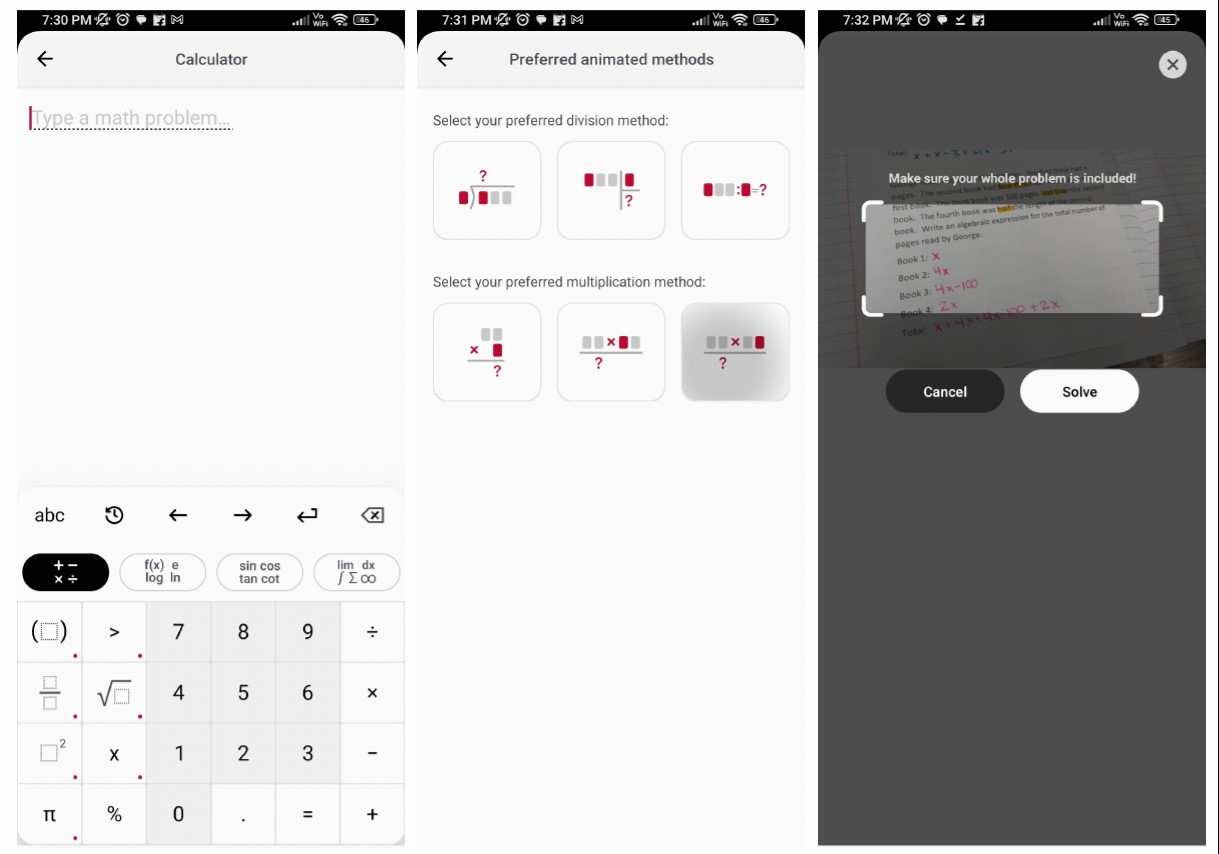Photomath is a groundbreaking Android app you can use to solve doubtful math problems. The app uses augmented reality technology to detect math questions through your camera and solve them for you. You may scan handwritten or printed maths problems and Photomath will provide comprehensive, step-by-step answers. Moreover, the app also provides a thorough explanation of the answers enclosing the ability to grasp fundamentals as well. Photomath makes sure that no math issue is too difficult by covering a wide variety of math topics. Users of the app may learn more about mathematical ideas by learning each step as it leads to the answer. In conclusion, Photomath makes maths learning fun and interesting with animated tutorials and dynamic graphs

## Microsoft Math Solver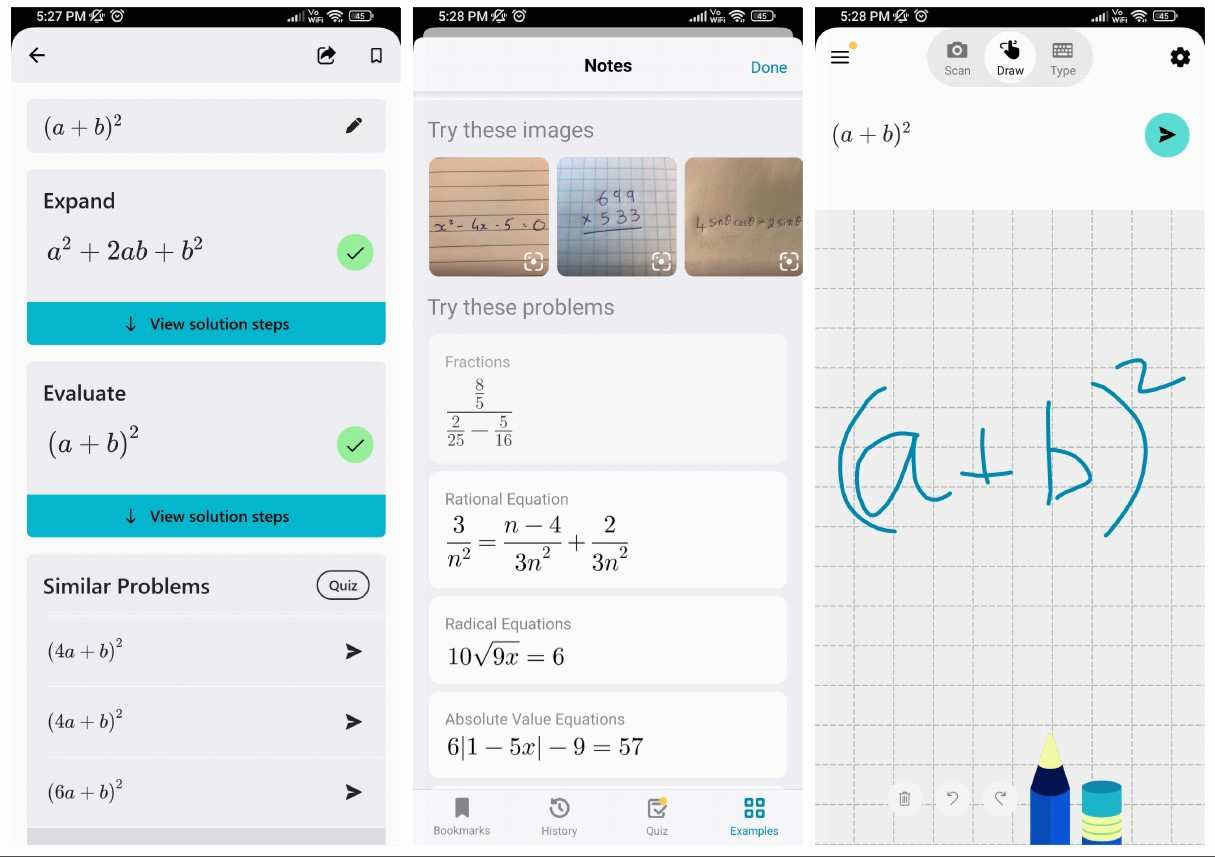Microsoft Math Solver is very similar to Photomath in terms of its ability to solve intricate math problems. This app from Microsoft is a great math solver app that caters to the various learning demands of students. This app can help you whether you’re working with simple algebraic formulas or trigonometric equations. Moreover, It can scan handwritten maths problems or manually input equations. You can draw a rough equation of the math problem and Microsoft Math Solver will be able to read it. The accuracy is always precise and gives a proper solution all the time. In addition, it also provides thorough, step-by-step explanations, enabling users to completely comprehend each solution. Microsoft Math Solver has an interactive graph that enables the door for students to learn in new ways. They can visualise the functions and better understand mathematical ideas. Microsoft Math Solver also provides interactive quizzes and unit conversions to support learners.

## Gauthmath Expert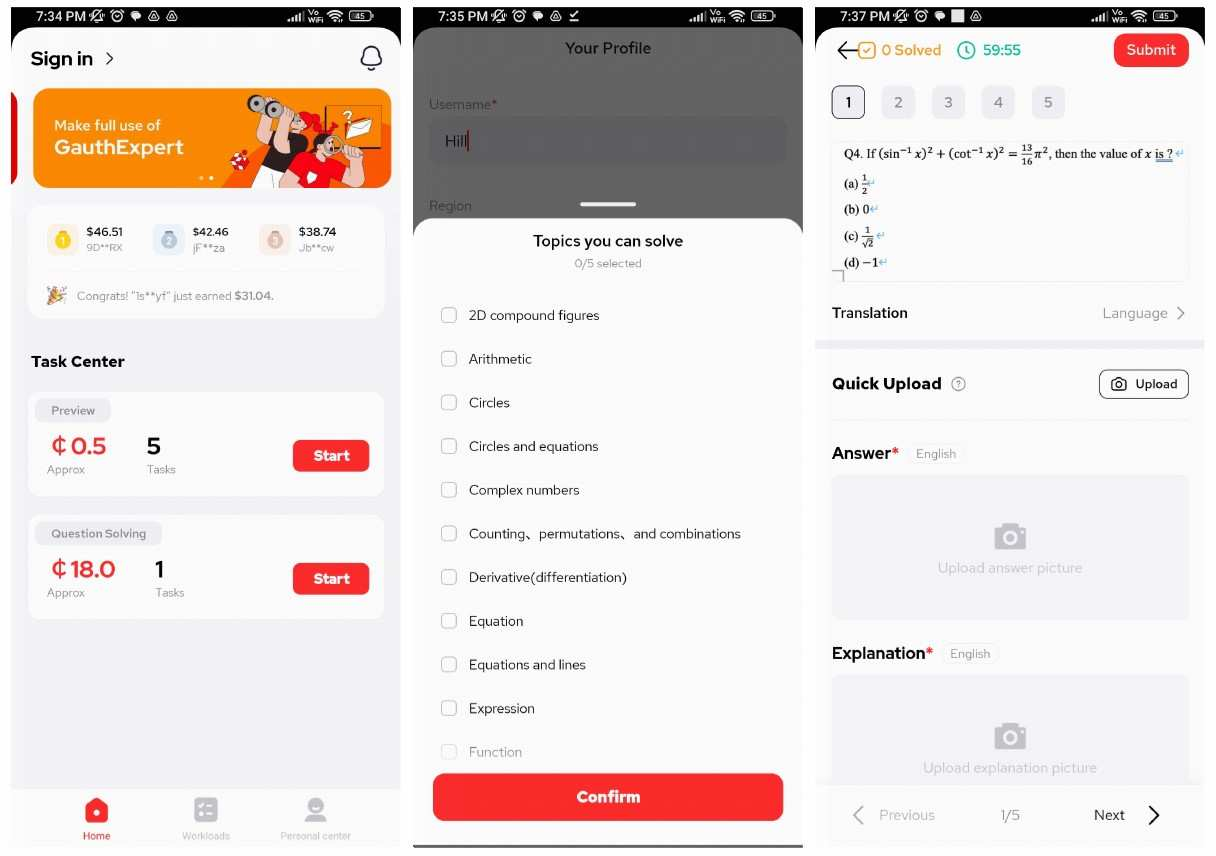Gauthmath Expert is a flexible math-solving tool that utilizes your camera to do its operation. It is a great companion for students and learners of various skill levels. This app offers a wide range of maths subjects that you learn as a student. As a result, one can also call it a portable tutor. The app also has a camera-based feature that reads the equations that you draw on the board. Beyond providing speedy solutions, Gauthmath Expert offers thorough step-by-step explanations, ensuring that students are learning along with finding solutions. The software helps to increase problem-solving abilities and reinforce learning with individualised quizzes and practice exercises.

## Mathway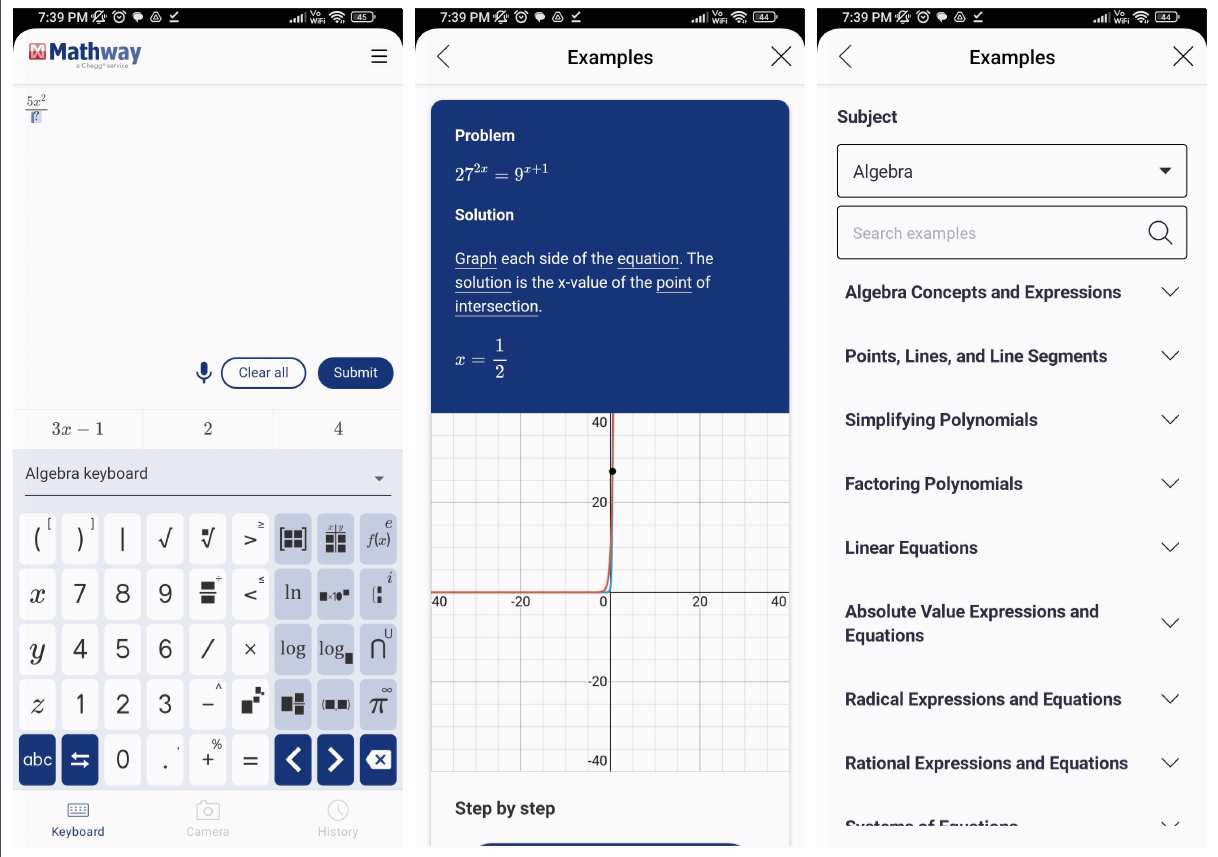Mathway stands out as a quick and adaptable math-solving app that can figure out various levels of math problems. There are three ways to input your doubts in Mathway and they are typing, scanning, or voice commands. Mathway covers multiple mathematical topics such as algebra, calculus, trigonometry, and others. However, users have to pay for subscriptions to enjoy a thorough explanation of answers. When dealing with problems that must be solved quickly, Mathway’s clever algorithms quickly produce correct solutions. Graphs, charts, and useful formulas present in Mathway are what makes it a distinct math-solving app that genuinely sets it apart from other study tools.

## CameraMath – Homework Help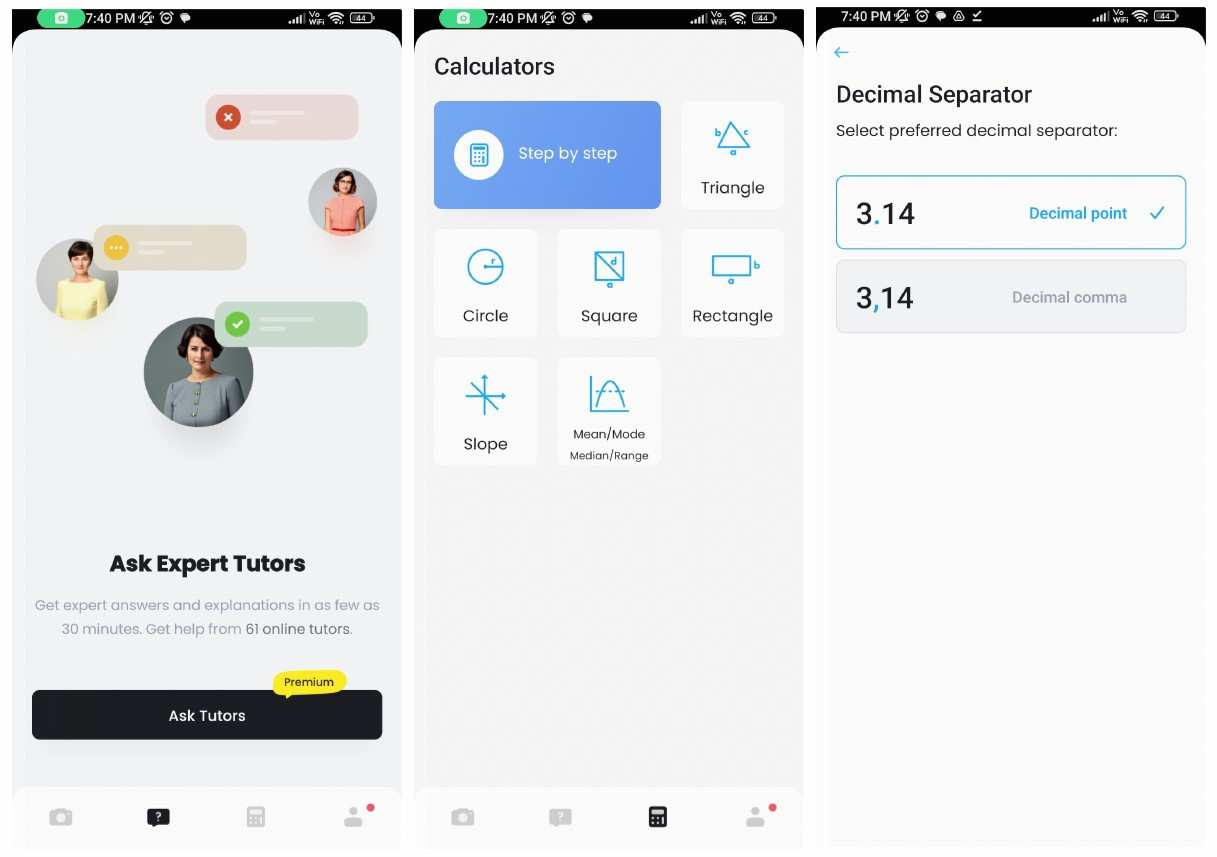Another math solver with an image recognition feature in our list is the CameraMath. It is a priceless math-solving software that excels at delivering answers to mathematical puzzles using the camera on your device. You get a step-by-step explanation of their maths problems using AI to extract key information. For students who want immediate help with their tasks, the app’s sole focus on homework aid makes it very useful. Additionally, CameraMath has a clever calculator that supports a number of math operations, such as arithmetic, trigonometry, and logarithms. CameraMath is a popular option among students because of its interactive features and user-friendly layout. As a result, it encourages students to explore and do challenging maths questions with ease.

## Conclusion

Students can have a hard time grasping the complexities of math concepts. With these intuitive and educational tools, they can learn math in a new and better way. While these apps may not replace the need for a human tutor, they are certainly a great addition to the lives of hardworking students. All the apps are easily available and you can quickly install and start using them.